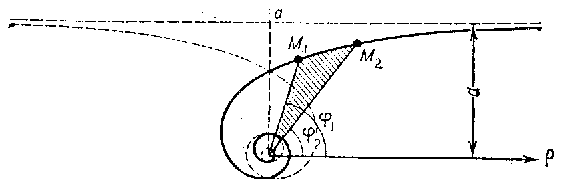# Hyperbolic spiral

A plane transcendental curve whose equation in polar coordinates is

$$\rho=\frac a\phi.$$

It consists of two branches, which are symmetric with respect to a straight line $d$ (see Fig.). The pole is an asymptotic point. The asymptote is the straight line parallel to the polar axis at a distance $a$ from it. The arc length between two points $M_1(\rho_1,\phi_1)$ and $M_2(\rho_2,\phi_2)$ is

$$l=a\left[-\frac{\sqrt{1+\phi^2}}{\phi}+\ln(\phi+\sqrt{1+\phi^2})\right]_{\phi_1}^{\phi_2}.$$

The area of the sector bounded by an arc of the hyperbolic spiral and the two radius vectors $\rho_1$ and $\rho_2$ corresponding to the angles $\phi_1$ and $\phi_2$ is

$$S=\frac{a^2(\rho_1-\rho_2)}{2}.$$

A hyperbolic spiral and an Archimedean spiral may be obtained from each other by inversion with respect to the pole $O$ of the hyperbolic spiral.Figure: h048340a

A hyperbolic spiral is a special case of the so-called algebraic spirals.